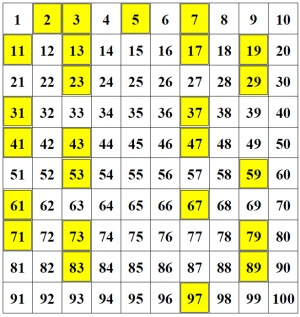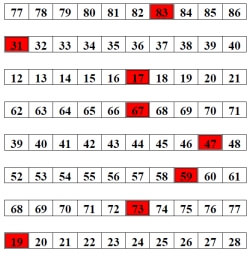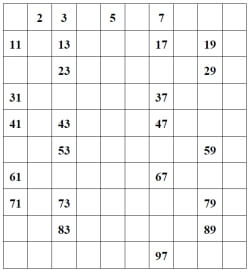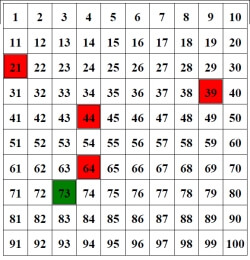Worksheets and No Prep Teaching Resources Math WorksheetsPrime Numbers and Prime Factorization Activities, Worksheets, Printables, and Lesson Plans

Prime NumbersFill-in all of the prime numbers up to 100 Color in the prime numbers up to 100 (5 are given) Color in the prime numbers up to 100 (2 are given) Rows - Color in the prime numbers (1 given in each row) Rows - Color in the prime numbers

Composite NumbersFill-in all of the prime numbers up to 100 Color in the prime numbers up to 100 (5 are given) Color in the prime numbers up to 100 (2 are given) Rows - Color in the prime numbers (1 given in each row) Rows - Color in the prime numbers

Prime or Composite NumbersColor in the prime numbers up to 100 (5 are given) Color in the prime numbers up to 100 (2 are given) Rows - Color in the prime numbers (1 given in each row) Rows - Color in the prime numbers

Number Theory
Prime or composite
Divisibility: complete the table
Divisibility: fill in the missing digit
List all of the factors
Prime factorization
Greatest common factor
Least common multiple
Open-ended number theory questions
Number theory review printable

Have a suggestion or would like to leave feedback?